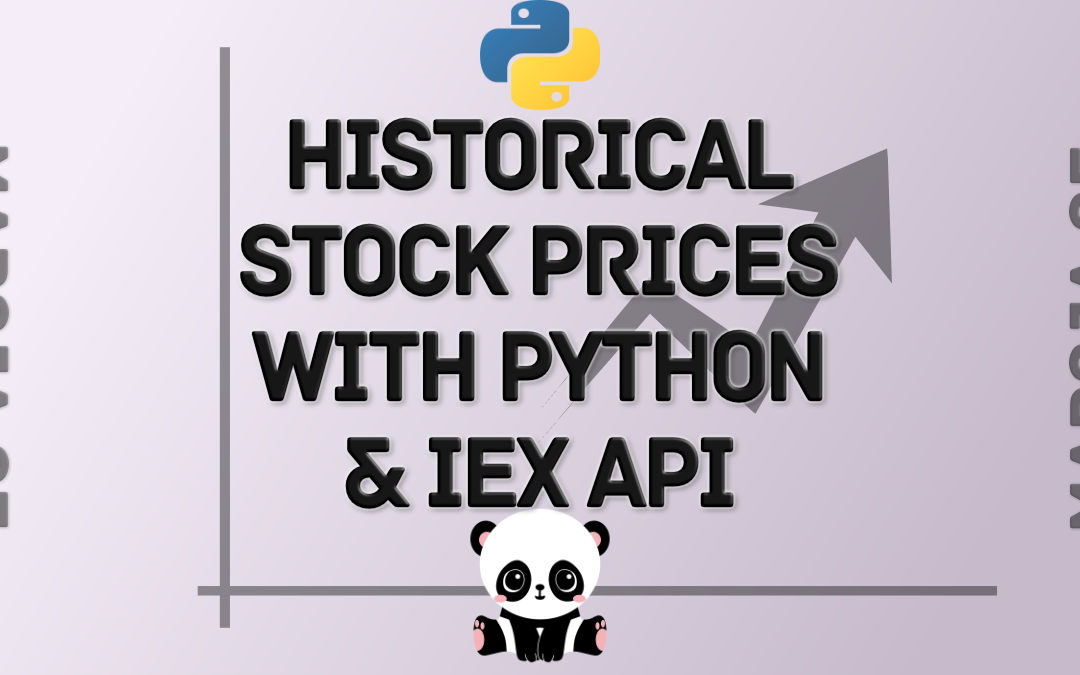## How to Import Historical Stock Prices Into A Python Script Using the IEX Cloud API

Python is one of the world’s most popular programming languages. Specifically, Python for finance is arguably the world’s most popular language-application pair. This is because of the robust ecosystem of packages and libraries that makes it easy for...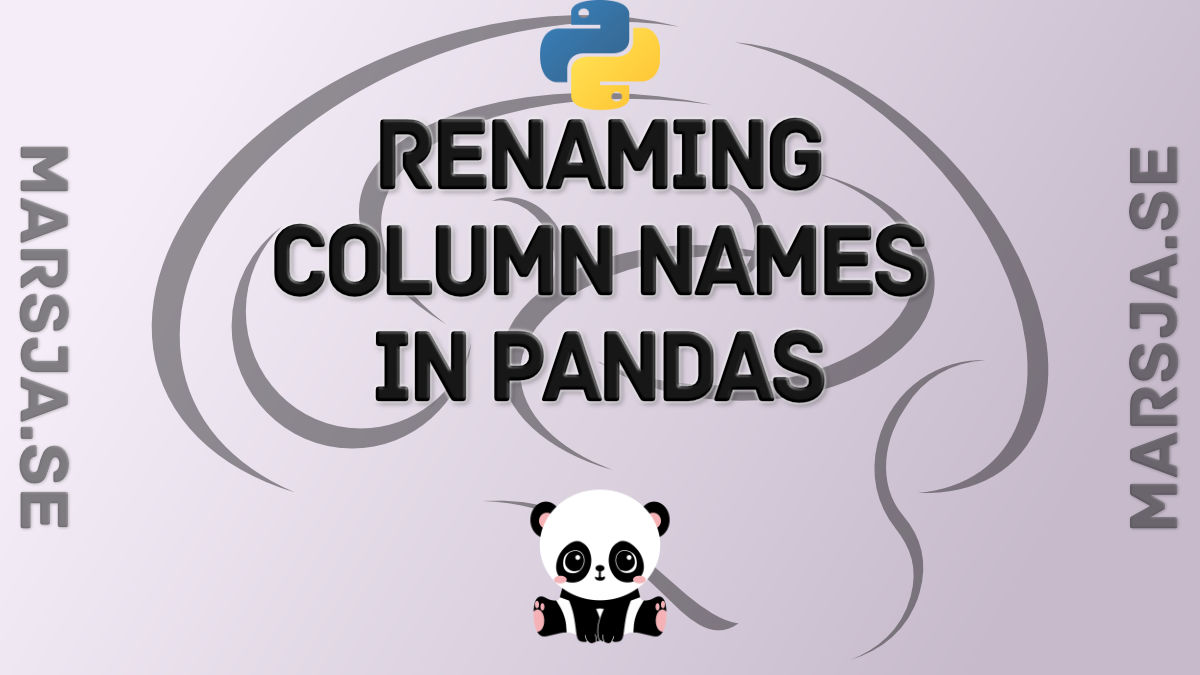## Pandas Tutorial: Renaming Columns in Pandas Dataframe

In this Pandas tutorial, we will go through how to rename columns in a Pandas dataframe. First, we will learn how to rename a single column. Second, we will go on with renaming multiple columns. In the third example, we will also have a quick look at how to rename...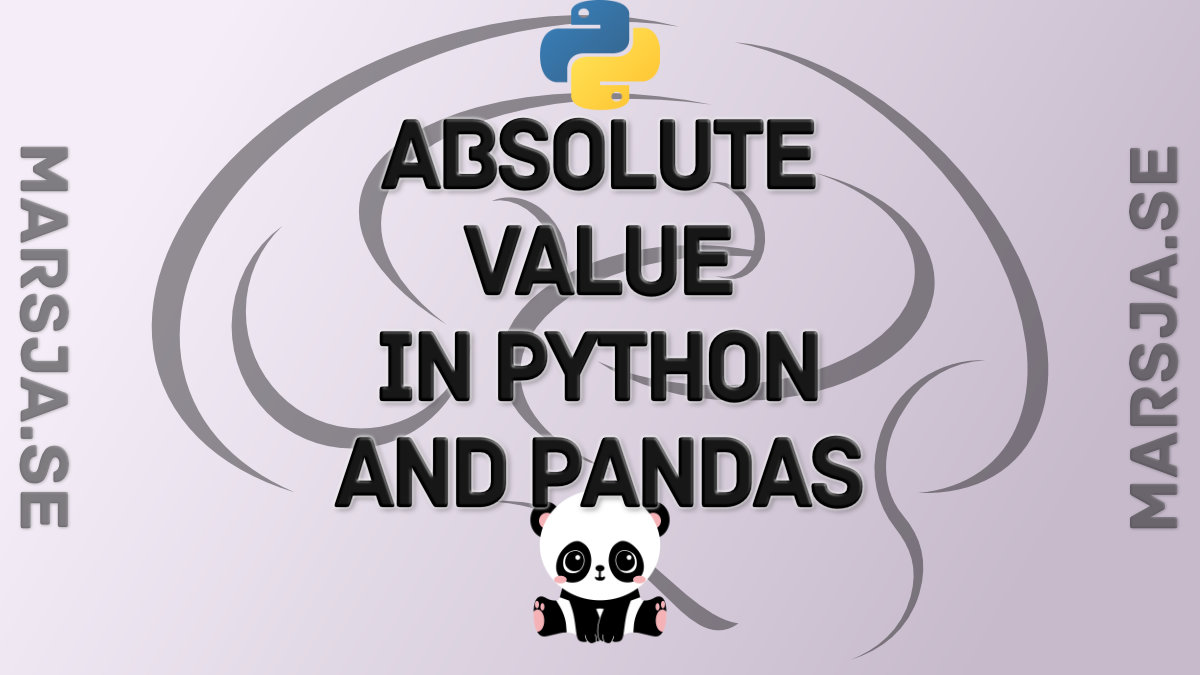## How to get Absolute Value in Python with abs() and Pandas

In this Python tutorial, we will learn how to get the absolute value in Python. First, we will use one of Pythons built-in functions abs() to do this. In this section, we will go through a couple of examples of how to get the absolute value. Second, we will import...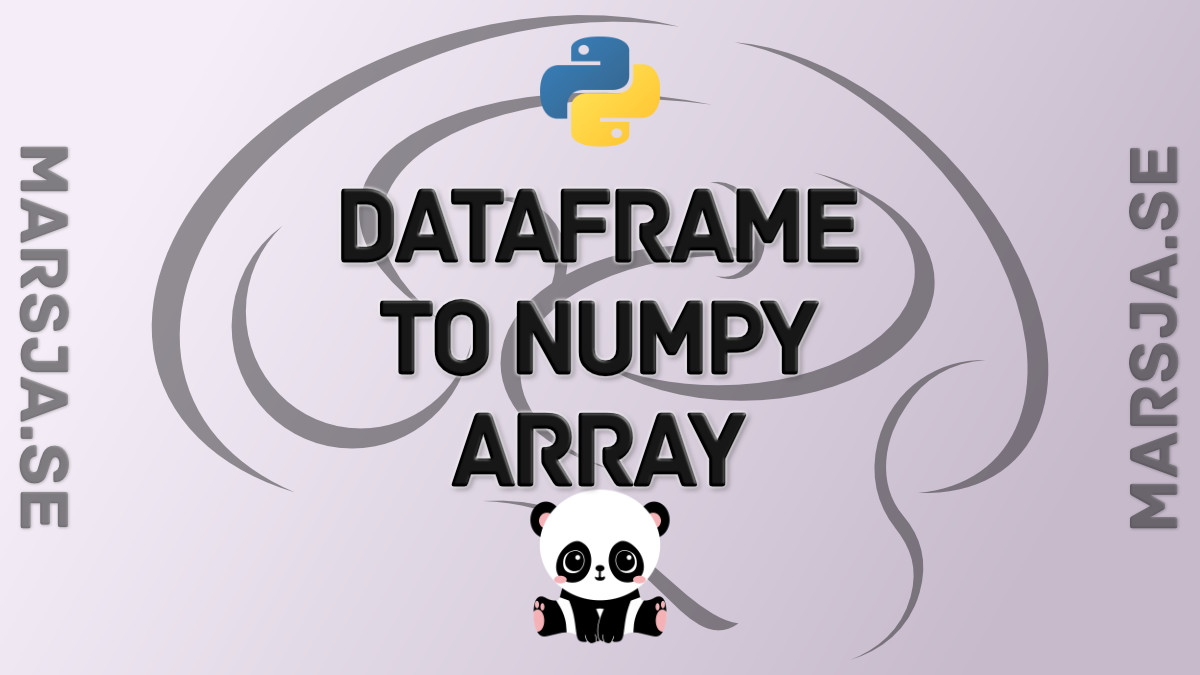## How to Convert a Pandas DataFrame to a NumPy Array

In this short Python Pandas tutorial, we will learn how to convert a Pandas dataframe to a NumPy array.  Specifically, we will learn how easy it is to transform a dataframe to an array using the two methods values and to_numpy, respectively. Furthermore, we will...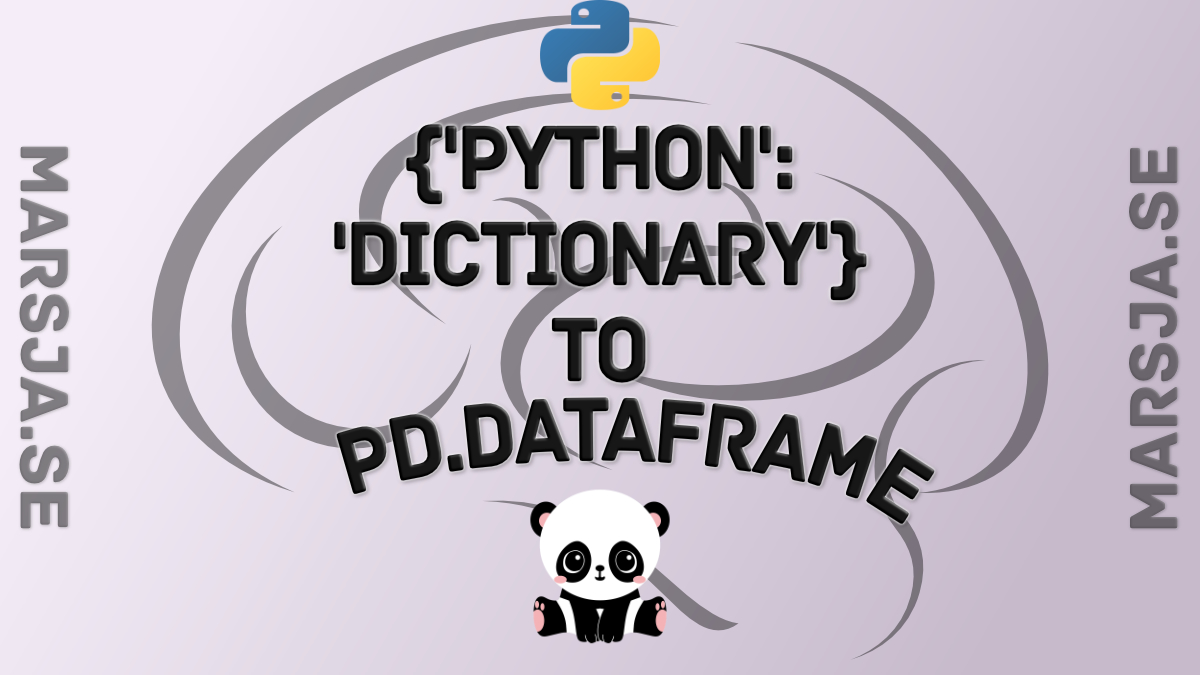## How to Convert a Python Dictionary to a Pandas DataFrame

In this brief Python Pandas tutorial, we will go through the steps of creating a dataframe from a dictionary. Specifically, we will learn how to convert a dictionary to a Pandas dataframe in 3 simple steps. First, however, we will just look at the syntax. After we...